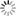Episode List

OR

Unknown Season

1999
The Films of Barry SonnenfeldKnow what this is about?
Be the first one to add a plot.

1999
The Films of Brett Ratner
7.2Know what this is about?
Be the first one to add a plot.

1999
The Films of John AdvildsenKnow what this is about?
Be the first one to add a plot.

1999
The Films of John Carpenter
7.8 (6)Know what this is about?
Be the first one to add a plot.

1999
The Films of John SingletonKnow what this is about?
Be the first one to add a plot.

1999
The Films of Lasse HallstromKnow what this is about?
Be the first one to add a plot.

1999
The Films of Mike FiggisKnow what this is about?
Be the first one to add a plot.

1999
The Films of Phillip NoyceKnow what this is about?
Be the first one to add a plot.

1999
The Films of Sidney Lumet
6.5 (10)Know what this is about?
Be the first one to add a plot.

1 Mar. 1999
The Films of Norman Jewison
5.9 (25)Know what this is about?
Be the first one to add a plot.

5 Mar. 1999
The Films of Shekhar KapurKnow what this is about?
Be the first one to add a plot.

9 Jul. 1999
The Films of Lars Von TrierKnow what this is about?
Be the first one to add a plot.

17 Dec. 1999
The Films of John L'EcuyerKnow what this is about?
Be the first one to add a plot.

17 Mar. 2000
The Films of Vincenzo NataliKnow what this is about?
Be the first one to add a plot.

5 May 2000
The Films of François GirardKnow what this is about?
Be the first one to add a plot.

26 May 2000
The Films of Don McKellarKnow what this is about?
Be the first one to add a plot.

16 Jun. 2000
The Films of Denys ArcandKnow what this is about?
Be the first one to add a plot.

5 Oct. 2000
The Films of Wolfgang Petersen
7.8 (23)German director Wolfgang Petersen achieved international recognition with his 1981 film "Das Boot", and has since conquered Hollywood with such hits as "The Neverending Story", "In the Line of Fire", "Outbreak", "Air Force One", and "The Perfect Storm". In this film some of the stars he has worked with including Diane Lane, Dustin Hoffman, Harrison Ford and Rene Russo talk about his work, the director and the man.

2001
The Films of Penny MarshallKnow what this is about?
Be the first one to add a plot.

2001
The Films of Robert LuketicKnow what this is about?
Be the first one to add a plot.

9 Feb. 2001
The Films of Garry Marshall
7.5 (11)Know what this is about?
Be the first one to add a plot.

5 May 2001
The Films of Lawrence Kasdan
6.8 (6)Know what this is about?
Be the first one to add a plot.

1 Aug. 2001
The Films of Derek LambKnow what this is about?
Be the first one to add a plot.

24 Aug. 2001
The Films of Lea PoolKnow what this is about?
Be the first one to add a plot.

2003
Macauly CulkinKnow what this is about?
Be the first one to add a plot.

2005
The Films of Kevin Smith
6.6 (14)Know what this is about?
Be the first one to add a plot.

7 Apr. 2005
The Films of Carl FranklinKnow what this is about?
Be the first one to add a plot.

2007
The Films of Adrian Lyne
7.4Know what this is about?
Be the first one to add a plot.

2008
The Films of Andy TennantKnow what this is about?
Be the first one to add a plot.

2008
The Films of David O. RussellKnow what this is about?
Be the first one to add a plot.

2008
The Films of Jan De BontKnow what this is about?
Be the first one to add a plot.

2008
The Films of Jon TurteltaubKnow what this is about?
Be the first one to add a plot.

The Best Moments
Today's top filmmakers speak about the profession - their beginnings, inspirations and challenges.

« Season 4 |  Unknown Season